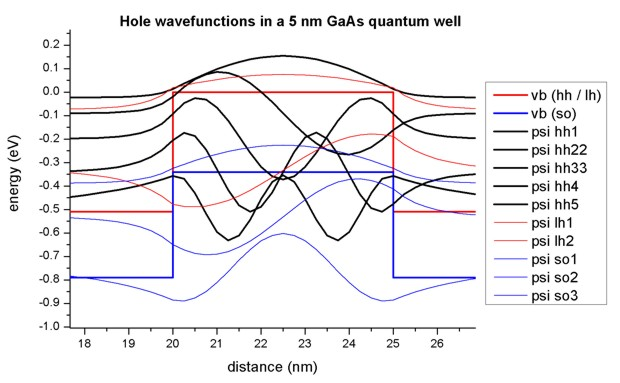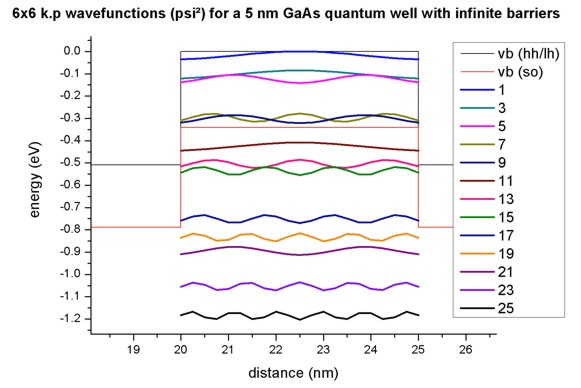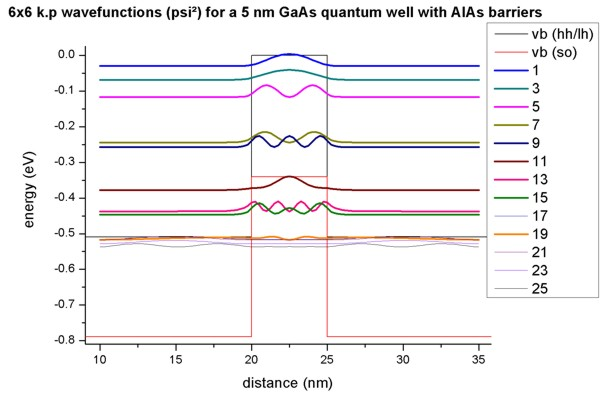nextnano.com  nextnano³  Download | Search | Copyright | Publications  * password protected nextnano³ software1D Interband Transitions

# nextnano3 - Tutorial

## Optical interband transitions in a quantum well - Matrix elements and selection rules

Author: Stefan Birner

```-> 1DQW_interband_matrixelements_finite_nn3.in       / *nnp.in - ```input file for the nextnano3 and nextnano++ software``` -> 1DQW_interband_matrixelements_infinite_nn3.in     / *nnp.in - ```input file for the nextnano3 and nextnano++ software``` -> 1DQW_interband_matrixelements_finite_kp_nn3.in -> 1DQW_interband_matrixelements_infinite_kp_nn3.in```

## Optical interband transitions in a 5 nm AlAs / GaAs / AlAs quantum well - Matrix elements and selection rules

(Note: This tutorial has to be updated: Now we output the square (!) of the matrix element` < psi_hl_i | psi_el_j >`, i.e. `< psi_hl_i | psi_el_j >^2`.)

### Eigenstates and wave functions in the quantum well

• We consider a 5 nm GaAs quantum well embedded between AlAs barriers. The structure is assumed to be unstrained.
We distinguish between two cases:
a) finite AlAs barriers
b) infinite "AlAs" barriers (This can be achieved by choosing Dirichlet boundary conditions at the quantum well boundaries.)
• Case a):

```-> 1DQW_interband_matrixelements_finite_nn3.in ```
For finite barriers we obtain using single-band Schrödinger effective-mass approximation (i.e. isotropic and parabolic effective masses)
- 3 confined electron states in the Gamma conduction band (we don't consider L and X bands here)
- 5 confined heavy hole states
- 2 confined light hole states
- 3 confined split-off hole states
The figure below shows the band edges of the Gamma conduction band and the heavy, light and split-off hole band edges together with wave functions of the confined states. Note that the heavy and light hole band edge is degenerate.• As one can see the valence band looks rather messy. Thus we zoom into it...
The 5 heavy hole wave functions are indicated in black, the 2 light hole wave function in red and the 3 split-off hole wave functions in blue.• Case b) Infinite AlAs barriers

```-> 1DQW_interband_matrixelements_infinite_nn3.in ```
To understand the optical transitions we first examine the matrix elements of the envelope functions, i.e. the spatial overlap which is the integral over their product with no dependence on polarization.

integral  (psicn* (z)  psivm (z) dz)

In our case, we have a symmetric quantum well, thus our envelope functions are either symmetric or antisymmetric. Therefore the matrix element will vanish unless the envelope functions have the same parity.

Now let's simulate the same structure as above but this time we assume infinite AlAs barriers:
Case b)``` -> 1DQW_interband_matrixelements_infinite.in ```In this case, the two sets of envelope functions are the same for both electron and hole wave functions and the integral becomes the Kronecker delta:

integral  (psicn* (z)  psivm (z) dz) = deltanm

This leads to the so-called 'Delta n = 0' selection rule, i.e. only transitions between levels with the same index are allowed. Of course, this rule is not valid any more for case a) where we have finite AlAs barriers but nevertheless this rule gives the strongest transitions.

``` \$output-1-band-schroedinger   ...   interband-matrix-elements = yes   complex-wave-functions    = yes ! ```to print out psi in addition to psi²``` ```
The spatial overlap integrals of the envelope functions are contained in these files:
```  Schroedinger_1band/interband1D_vb001_cb001_qc001_hlsg001_deg001_dir.dat - ```heavy hole```   Schroedinger_1band/interband1D_vb002_cb001_qc001_hlsg002_deg001_dir.dat - ```light hole```   Schroedinger_1band/interband1D_vb003_cb001_qc001_hlsg003_deg001_dir.dat - ```split-off hole

For instance, the matrix elements of the envelope functions for the 'heavy hole' to 'conduction band' transitions read:
```   Spatial overlap matrix elements < psi_hl_i | psi_el_j > and                                           energy of transition in [eV].    heavy hole <-> Gamma conduction band --------------------------------------------------------   <psi_vb001|psi_cb001>  1.001844         1.729371   ```('Delta n = 0' selection rule)```   <psi_vb001|psi_cb002>  3.456436E-016   <psi_vb001|psi_cb003>  7.866970E-016   <psi_vb002|psi_cb001>  7.463647E-016   <psi_vb002|psi_cb002>  1.007268         2.355209   ```('Delta n = 0' selection rule)```   <psi_vb002|psi_cb003>  2.844946E-016   <psi_vb003|psi_cb001>  9.575673E-016   <psi_vb003|psi_cb002>  1.450228E-015   <psi_vb003|psi_cb003>  1.015938         3.384106   ```('Delta n = 0' selection rule)```   <psi_vb004|psi_cb001>  1.076395E-015   <psi_vb004|psi_cb002>  1.422473E-015   <psi_vb004|psi_cb003>  2.019218E-015   <psi_vb005|psi_cb001>  1.960237E-016   <psi_vb005|psi_cb002>  1.346145E-015   <psi_vb005|psi_cb003>  1.217775E-015 ```The results shown above are for a 0.25 nm grid spacing (which is rather coarse).
For a 0.1 nm gridding one obtains the following values for the relevant transitions:``` ``````  <psi_vb001|psi_cb001>  1.000140         1.754633   <psi_vb002|psi_cb002>  1.000559         2.459675   <psi_vb003|psi_cb003>  1.001251         3.631886  ```
• Case a) Finite AlAs barriers

We now calculate the same matrix elements as above but this time for the finite AlAs barriers.
```   Spatial overlap matrix elements < psi_hl_i | psi_el_j > and                                           energy of transition in [eV].    heavy hole <-> Gamma conduction band   -------------------------------------   <psi_vb001|psi_cb001>  0.987507         1.654103   ```('Delta n = 0' selection rule)```   <psi_vb001|psi_cb002>  1.336279E-014   <psi_vb001|psi_cb003>  0.145559         2.538366   ```(same parity: symmetric)```   <psi_vb002|psi_cb001>  1.133344E-014   <psi_vb002|psi_cb002>  0.964789         2.065139   ```('Delta n = 0' selection rule)```   <psi_vb002|psi_cb003>  7.879180E-015   <psi_vb003|psi_cb001>  0.128041         1.829856   ```(same parity: symmetric)```   <psi_vb003|psi_cb002>  4.286800E-015   <psi_vb003|psi_cb003>  0.839306         2.714118   ```('Delta n = 0' selection rule)```   <psi_vb004|psi_cb001>  6.263441E-015   <psi_vb004|psi_cb002>  0.215428         2.315853   ```(same parity: antisymmetric)```   <psi_vb004|psi_cb003>  1.246759E-015 ```
The results shown above are for a 0.25 nm grid spacing (which is rather coarse).
For a 0.1 nm gridding one obtains the following values for the relevant transitions:```   <psi_vb001|psi_cb001>  0.987955         1.652509   <psi_vb001|psi_cb003>  0.142978         2.541682   <psi_vb002|psi_cb002>  0.966524         2.062825   <psi_vb003|psi_cb001>  0.127100         1.828683   <psi_vb003|psi_cb003>  0.838394         2.717855   <psi_vb004|psi_cb002>  0.211786         2.317309  ```

### 6-band k.p calculations for the infinite barrier GaAs quantum well

`-> 1DQW_interband_matrixelements_infinite_kp_nn3.in`

The following figure shows the lowest 26 eigenstates obtained with 6-band k.p for the 5 nm GaAs quantum well with infinite barriers. Each k.p state is two-fold degenerate (spin up / spin down).One can easily relate the transitions to the 'Delta n = 0' selection rule. However, in contrast to the single-band approximation, the matrix elements are not necessarily equal to 1 any more because the hole states are mixed and thus the hole envelope functions are significantly different to the electron envelope functions, even for an infinitely deep square well.

```   Spatial overlap matrix elements < psi_hl_i | psi_el_j > and                                           energy of transition in [eV].    6-band k.p holes <-> Gamma conduction band   --------------------------------------------------------    ...  ```

### 6-band k.p calculations for the finite barrier AlAs/GaAs/AlAs quantum well

`-> 1DQW_interband_matrixelements_finite_kp_nn3.in`

• The following figure shows the 6-band k.p hole wave functions for the quantum well having finite AlAs barriers. Their energies and psi² are two-fold degenerate due to spin but the wave functions psi are different! (not shown here)
The electron wave functions (3 confined states) are the same as above.• The file``` Schroedinger_kp/interband1D_vb001_cb001_qc001_hlsg001_deg001_dir.dat ```contains the following spatial overlap integrals.
One can nicely see that in addition to the transitions where the 'Delta n = 0' selection rule is responsible, additional transitions arise due to symmetric/antisymmetric parity. All other transitions are zero. This is in agreement with the single-band results.

```Electric field in z-direction [kV/cm]: 0.000000000000000E+000 Spatial overlap matrix elements < psi_hl_i | psi_el_j > and                                           energy of transition in [eV]. 6-band k.p holes <-> Gamma conduction band -------------------------------------------------------- ...```